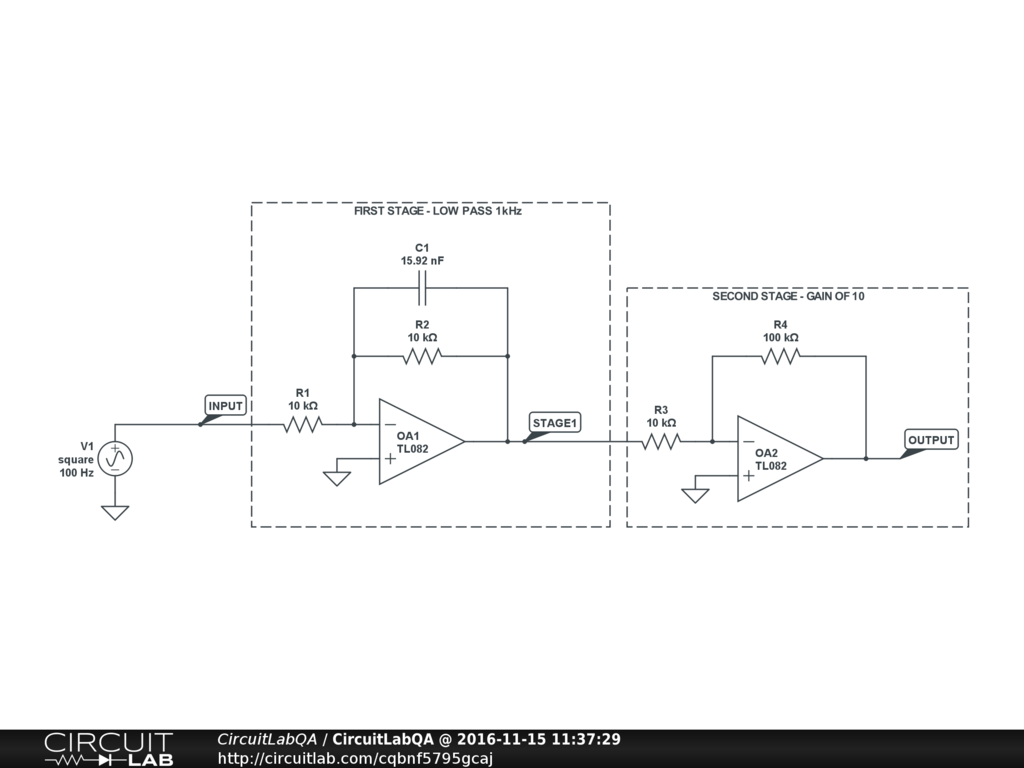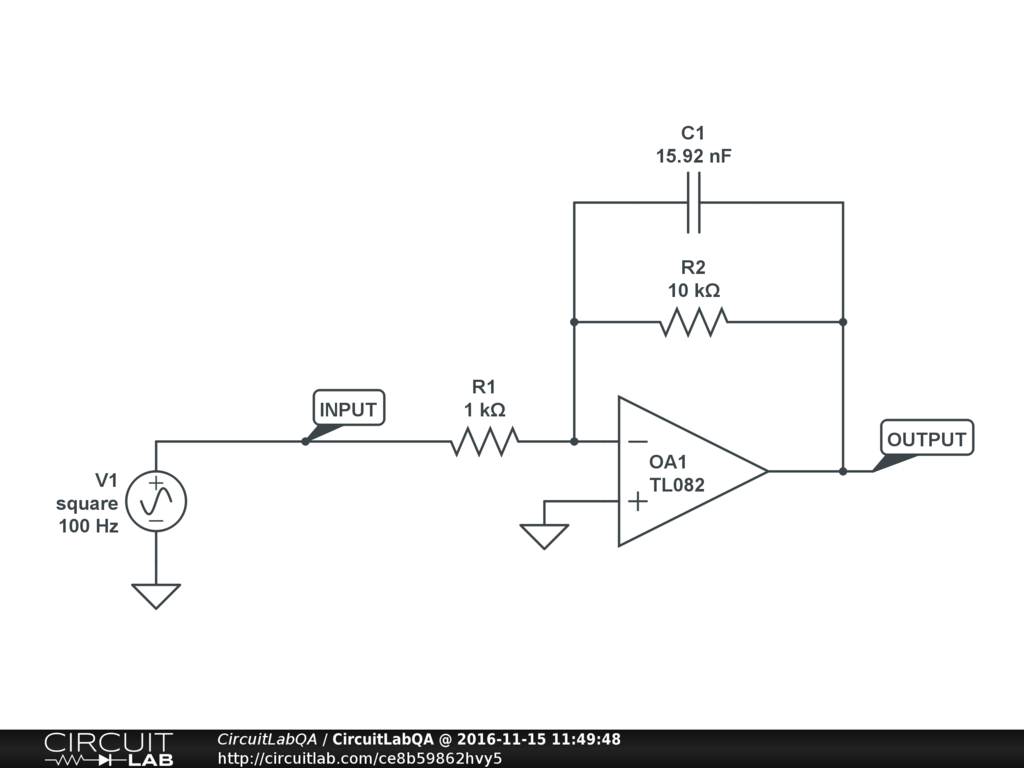## Design op-amp low-pass filter 1kHz gain of 10 SOLVED

 Im supposed to show at least 2 different ways to make a low pass filter using op amps, capacitors, resistors. Corner 1000 hertz and gain of 10. The homework question is show how to do it with 1 op amp or with 2 op amps. Why are these different? by CAMIE538 November 15, 2016 Does the gain have to be +10 or can it be inverting gain of -10? by berniekorrie November 15, 2016 either one by CAMIE538 November 15, 2016

 Here's a way to do it with two. First opamp you can do the low-pass part, and second you can do the gain. For the first one, say we pick $R_1 = R_2 = 10k\Omega$. We can just pick it as long as its reasonable for the rest of the circuit. Then for the capacitor we have $$\tau = R_1 C_1 = \frac {1} {2 \pi f_c}$$ with $f_c = 1000$, so $$C_1 = \frac {1} {2 \pi \times 1000 Hz \times 10k\Omega}$$ $$C_1 = 15.9 nF$$ For the second one we just do an inverting gain of 10, so maybe $R_3=10k\Omega$ and $R_4=100k\Omega$. Here it is:Click it, try the frequency domain simulation, look at the plot. It has +20dB gain at DC - that's a gain of 10 for all you non-decibel-speaking folks. It rolls off with the -3dB point (-3dB relative to the flat area, so the plot says +17dB) exactly at 1kHz. Please accept my answer! ACCEPTED +2 votes by berniekorrie November 15, 2016

 OK I copy+pasted berniekorrie's circuit and made it work with just one opamp. copy+paste berniekorrie 2 stage circuit deleted second stage changed $R_1$ from $10k\Omega$ to $1k\Omega$ Click it and run the bode plot, it works! Only difference is the phase: now it's 180 degrees at DC because it's inverting. Doesnt have 2nd stage to invert it back:+1 vote by CAMIE538 November 15, 2016

CircuitLab's Q&A site is a FREE questions and answers forum for electronics and electrical engineering students, hobbyists, and professionals.

We encourage you to use our built-in schematic & simulation software to add more detail to your questions and answers.

Acceptable Questions:

• Concept or theory questions
• Practical engineering questions
• “Homework” questions
• Software/hardware intersection
• Best practices
• Design choices & component selection
• Troubleshooting

Unacceptable Questions:

• Non-English language content
• Non-question discussion
• Non-electronics questions
• Vendor-specific topics
• Pure software questions
• CircuitLab software support

Please respect that there are both seasoned experts and total newbies here: please be nice, be constructive, and be specific!